# (Paper) CBSE Class XII : Chemistry Exam Paper Year 2009 (ISC Board)

Disclaimer: This website is not at associated with CBSE, For official website of CBSE visit - www.cbse.nic.in

## CBSE Class XII : Chemistry Exam Paper Year 2009 (ISC Board)

General Instruction:
(i). All working including rough work should be done on the same sheet as, and adjacent to the rest of the answer.
(ii). The intended marks for questions or parts of questions are given in brackets []. (Material to be supplied: Log tables including Trigonometric functions)
(iii). A list of useful physical constants is given at the end of this paper.

## PART I

Q1. (a) Correct the following statements by changing the underlined part of the sentence. (Do not change the whole sentence) : 

(i) For all spontaneous processes the change in entropy is zero.
(ii)
Formaldehyde undergoes Cannizzaro’s reaction since it has one alpha hydrogen atom.
(iii)
In a co-ordination complex, donation of electron pair takes place from the central metal atom to the ligands.
(iv)
An aqueous solution of sodium sulphate is acidic in nature.
(v)
The solubility product of sliver chloride in water decreases on addition of potassium chloride to the solution.

(b) Complete the following statements by selecting the correct alternative from the choices given: 

(i) The number of Faradays required to reduce one mol of (Cu)+2 to metallic copper is:
(a) One (b) Two
(c) Three (d) Four.

(ii) When oxalic acid is heated with glycerol we get:
(a) Formic acid (b) Acetic acid
(c) Lactic acid (d) Tartaric acid.

(iii) The molecular weight of sodium chloride determined by measuring the osmotic pressure of its aqueous solution is:
(a) Double the theoretical value (b) Same as the theoretical value
(c) Half the theoretical value (d) Three times the theoretical value.

(iv) In ethyne molecule there are:
(a) Only three sigma bonds
(b) Only three pi bonds
(c) Three sigma bonds and two pi bonds
(d) Three pi bonds and two sigma bonds.

(v) An example of an electrophil is:
(a) NO2+ (b) NO2-
(c) NO2 (d) NO3-

(c) Fill in the blanks by choosing the appropriate word / words from those given in the brackets : 
(paramagnetic, ethanol, diethyl ether, electron, proton, neutron, directly, Glucose, one, two depression, inversely, Fructose, Lewis acid, Lewis base, positive, ions, diamagnetic, negative, two, molecules, elevation, atoms)
(i) ………. and ……. are functional isomers.
(ii) AICl3 is a ……… because it is an ……………. deficient molecule.
(iii) The ……………. of the boiling point of a solvent by the addition of a solute is ……. proportional to the molality of the solution.
(iv) The crystal of diamond is made of ………….. while that of calcium chloride is made of ……… .
(v) Oxygen molecule is………… due to the presence of …………… unpaired electrons.

(d) Match the following:

 (i) Co-ordination compounds (ii) Dilute solution (iii) Electrolysis (iv) Toluene (v) Radioactivity (a) Raoult’s law (b) Group displacement law (c) Werner’s theory (d) Faraday’s law (e) Friedel Craft’s reaction.

## PART II (SECTION A)

Q2:
(a) (i)
The boiling point of pure water is 373K. Calculate the boiling point of an aqueous solution containing 18 gms of glucose (MW = 180) in 100 gms of water. Molal elevation constant of water is 0.52 K kg/mol. 

(ii) Equal weights of two substances X and Y are dissolved in equal volumes of water. The osmotic pressure of the solution containing Y is five times the osmotic pressure of the solution containing X. What is the molecular weight of X if that of Y is 60?

(iii) Which of the following solutions will have a lower vapour pressure and why?
(1) A 5% solution of cane sugar (C12H22O11)
(2) A 5% solution of urea (NH2CO NH2)
(Relative atomic masses: H = 1, C = 12, 0 = 16, N = 14)

(b) (i) When ammonium chloride and ammonium hydroxide are added to a solution containing both (Al)+3 and (Ca)+2 ions, which ion is precipitated? Why? 
(ii)
Explain why a solution of copper chloride is acidic while that of sodium chloride is neutral.

Q3. (a) (i) In a body centred and face centred arrangement of atoms of an element, what will be the number of atoms present in respective unit cells? 
(ii)
Explain why graphite is soft and can be used an a lubricant.
(b)
1.0 gm. of strontium – 90 was reduced to 0.953 gm after two years. Calculate the half life period of strontium – 90. 
(c)
Mention any two factors affecting the electrode potential of a metal. 
(d)
Draw the electron-dot or equivalent structure of perchloric acid. 

Q4. (a) What is the hybridisation of the central atom in ammonia, water and methane? Arrange them in the order of increasing bond angle. Give reasons. 

(b) Name the type of isomerism shown by the following pairs of co-ordination compounds: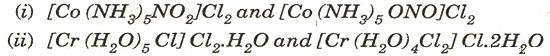(c) For the following cell, calculate the emf: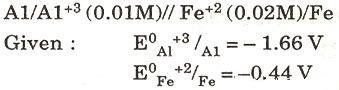(d) Mention one use each of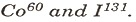## SECTION B (Answer any two questions)

Q 5:
(a) (i)
Write the mathematical expression for the first law of thermodynamics. One mole of an ideal gas is expanded isothermally against a constant pressure of 3 atmospheres from 10 litres to 35 litres. Calculate the work done, change in internal energy and heat absorbed during the process. 

(ii) Calculate the free energy change of the reaction: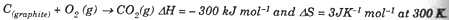Predict whether the reaction is spontaneous or not at 300 K

(b) An alkyl bromide undergoes reaction in the alkaline medium to form the corresponding alcohol. When the concentration of the alkyl bromide is doubled keeping the concentration of the alkali constant, the rate of the reaction is doubled. When the concentration of the alkali is doubled keeping the concentration of the alkyl bromide constant, the rate of the reaction remains the same.Write the mechanistic steps for the reaction and state the type of the reaction and the nature of the reagent. 

Q6. (a) Describe the extraction of silver from its sulphide ore by the cyanide process. 
(b)
Arrange the following in increasing order of acidity and explain your order:
Formic acid, acetic acid, chloroacetic acid. 

Q7. (a) How can crystalline sodium thiosulphate be prepared starting from sulphur ? What particular property of sodium thiosulphate is responsible for its use as an antichlor in the textile industry? 
(b)
Write balanced equations for each of the following reactions: 
(i) Fluorine and dilute sodium hydroxide.
(ii) Ozone and hydrogen sulphide.
(iii) Hydrogen peroxide and sodium hydroxide.

(c) What are alums? Give two uses of potash alum. 

## SECTION C (Answer any two questions)

Q8-:(a) How will you bring about the following conversions : 
(i)
Acetaldehyde to acetamide.
(ii)
Phenol to benzoic acid
(iii)
Glucose to saccharic acid.
(b)
Give the names of the following reactions: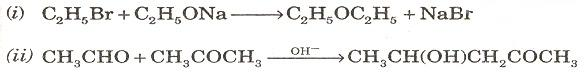Q9. (a) How will you distinguish between the following pairs of compounds? (Give one good chemical test.) 
(i) Ethylamine and Acetamide
(ii) Oxalic acid and Acetic acid

(b) An Organic compound A with molecular formula C2H7N on reaction with nitrous acid give a compound B. B on controlled oxidation gives a compound C. C reduces Tollen’s reagent to give silver mirror and D. B reacts with D in the presence of concentrated sulphuric acid to give a sweet smelling compound E. Identify A, B, C, D and E. Give the reaction of C with ammonia and name the product. 

(c) Draw the isomers of a compound with the molecular formula C4H4O4. Name the isomers. 

Q10 : (a) (i) What type of polymerisation takes place when a polyester is formed? Give one example of a polyester and name the monomers from which it is formed.
(ii) Give two examples of natural polymers.

(b) Identify the products A, B, C and D.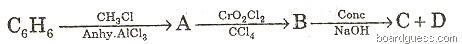(c) Give balanced equations for the following reactions:
(i) Formic acid heated with p’ollen’s reagent.
(ii) Phenol heated with chloroform and sodium hydroxide.
(iii) Acetaldehyde reacted with phenyl hydrazine.
(iv) Aniline treated with benzoyl chloride.

Disclaimer: This website is not at associated with CBSE, For official website of CBSE visit - www.cbse.nic.in

NEW!  Sample Papers Books : Class-X, Class-XII